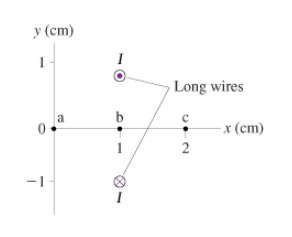# Problem: I = 8.0 AA. What is the magnetic field B at point a in the figure? Give your answer as vector.B. What is the magnetic field B at point b in the figure? Give your answer as vector.C. What is the magnetic field B at point c in the figure? Give your answer as vector.

###### FREE Expert Solution

To find the magnetic field, we'll use the equation for the magnetic field at a point due to a long straight wire given as:

$\overline{){\mathbf{B}}{\mathbf{=}}\frac{{\mathbf{\mu }}_{\mathbf{0}}\mathbf{i}}{\mathbf{2}\mathbf{\pi }\mathbf{r}}}$

86% (440 ratings)###### Problem DetailsI = 8.0 A

A. What is the magnetic field B at point a in the figure? Give your answer as vector.

B. What is the magnetic field B at point b in the figure? Give your answer as vector.

C. What is the magnetic field B at point c in the figure? Give your answer as vector.